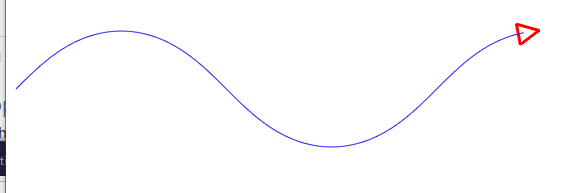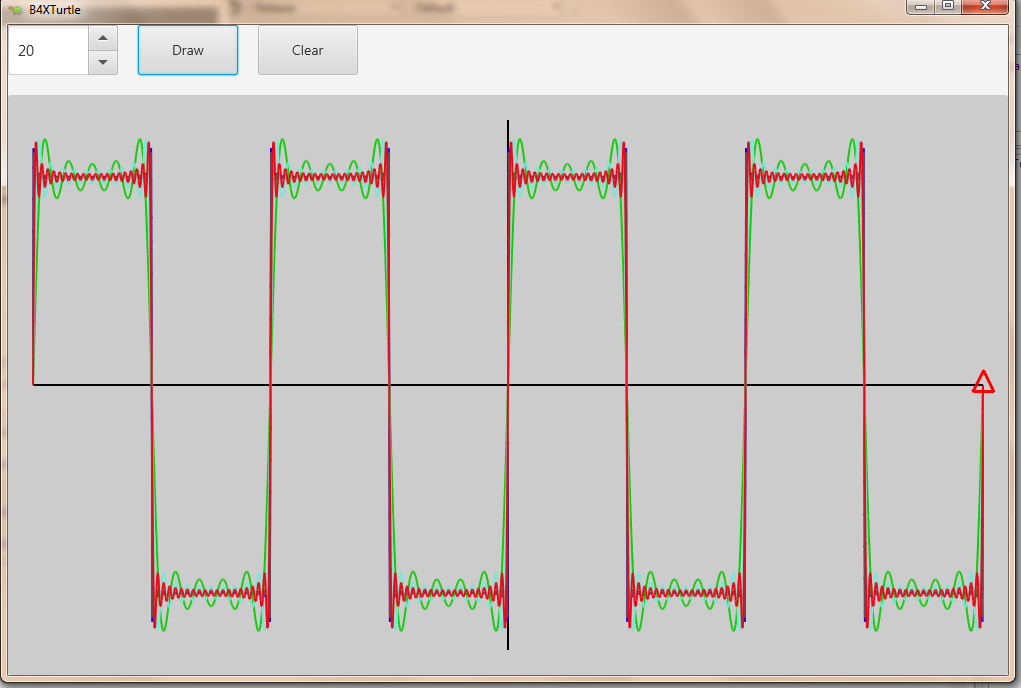# 🐢 B4XTurtle - Examples for teachers and parents

#### Erel

##### B4X founder
Staff member
Longtime User
The sine function has a very interesting characteristic - its second derivative equals to -sine(x).
With this knowledge we can draw it with very simple code and without using any trigonometric function.
B4X:
``````Sub Turtle_Start
Turtle.RabbitMode.SetPenColor(xui.Color_Blue).SetPenSize(1)
Dim YZero As Float = 100
Turtle.SetY(YZero).SetX(10).SetAngle(-45)
For i = 1 To 600
Dim value As Float = Turtle.GetY - YZero 'f(x)
Turtle.TurnLeft(value * 0.01).MoveForward(1) 'TurnLeft = f''(x) * constant
Next
End Sub``````

It will only work in rabbit mode as we need to get the current position before executing the next command.#### Johan Schoeman

##### Expert
Longtime User
Using the Fourier Series.....
B4X:
``sin((2n−1)*x)/(2n−1)``
.... to generate a square wave.B4X:
``````#Region Project Attributes
#MainFormWidth: 1000
#MainFormHeight: 650
#End Region

#Region Form Preperation
'Template version 1.00
Sub Process_Globals
Private fx As JFX
Private MainForm As Form
Private xui As XUI 'ignore
Private Turtle As B4XTurtle
Dim zerox, zeroy, offset As Float

Private Spinner1 As Spinner
End Sub

Sub AppStart (Form1 As Form, Args() As String)
MainForm = Form1
MainForm.Show
Spinner1.SetNumericItems(1, 150, 1, 1)

End Sub

#End Region

Sub Turtle_Start

turtle_prep

End Sub

Private Sub btnDraw_Click

Dim val As Int = Spinner1.Value

Dim x As Float = 0
Dim y As Float = 0

Turtle.SetPenColor(xui.Color_ARGB(255, Rnd(0, 255), Rnd(0, 255), Rnd(0, 255)))
For i = -720 To 720
x = offset + (2 * zerox / (2 * cPI)) * ((i+720)/1440) * 2 * cPI            'add the offset to the calculated x position
y=0
For n = 1 To val                                                        'Fourier series for a square wave
y = y + (Sin((2*n-1) * i * 2 * cPI/360)/(2*n-1))
Next

y = offset + (y * -1 * zeroy) + zeroy

If i = -720 Then
Turtle.SetX(x)
Turtle.SetY(y)
End If
Turtle.MoveTo(x,y)
Next

End Sub

Private Sub btnClear_Click

Turtle.SetPenColor(xui.Color_Black)
Turtle.ClearScreen
turtle_prep

End Sub

Sub turtle_prep

offset = 25  'leave a gap of 25 at the top and bottom as well as on the left and right of the X and Y axis
zerox = (Turtle.Width - 2 * offset ) / 2
zeroy = (Turtle.Height - 2 * offset) / 2

Turtle.SetSpeedFactor(10)
Turtle.mBase.Color = xui.Color_LightGray                  'set the Turtle background color
Turtle.PenUp
Turtle.SetX(zerox + offset)
Turtle.SetY(0 + offset)
Turtle.PenDown
Turtle.MoveTo(zerox + offset, 2 * zeroy + offset)          'Draw the y-axis
Turtle.SetX(0 + offset)
Turtle.SetY(zeroy + offset)
Turtle.MoveTo(2 * zerox + offset , zeroy + offset)         'Draw the x-axis

End Sub``````

#### Attachments

• TurtleSquareWave.zip
4 KB · Views: 138
•Johan Hormaza and Erel